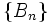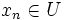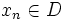# Second-countable implies separable

This article gives the statement and possibly, proof, of an implication relation between two topological space properties. That is, it states that every topological space satisfying the first topological space property (i.e., second-countable space) must also satisfy the second topological space property (i.e., separable space)
View all topological space property implications | View all topological space property non-implications

## Statement

### Property-theoretic statement

The property of topological spaces of being a second-countable space implies, or is stronger than, the property of being a separable space.

### Verbal statement

Every second-countable space is a separable space.

## Definitions used

### Second-countable space

Further information: Second-countable space

A topological space is termed second-countable if it admits a countable basis.

### Separable space

Further information: Separable space

A topological space is termed separable if it admits a countable dense subset.

## Proof

Given: A second-countable space$X$, with countable basis$\{ B_n \}$

To prove: There exists a countable dense subset of$X$

Proof: We can assume without loss of generality that all the$B_n$ are nonempty, because the empty ones can be discarded. Now, for each$B_n$, pick any element$x_n \in B_n$. Let$D$ be the set of these$x_n$s.$D$ is clearly countable (because the indexing set for its elements is countable). We claim that$D$ is dense in$X$.

To see this, let$U$ be any nonempty open subset of$X$. Then,$U$ contains some$B_n$, and hence,$x_n \in U$. But by construction,$x_n \in D$, so$D$ intersects$U$, proving that$D$ is dense.